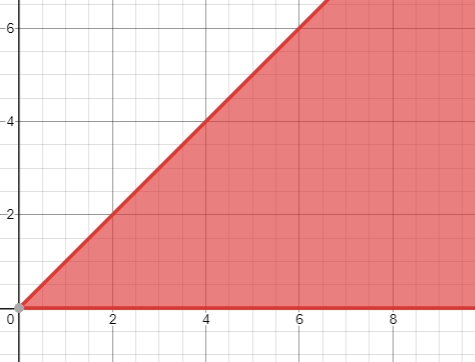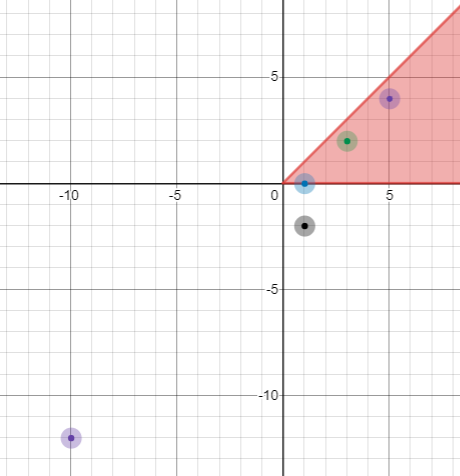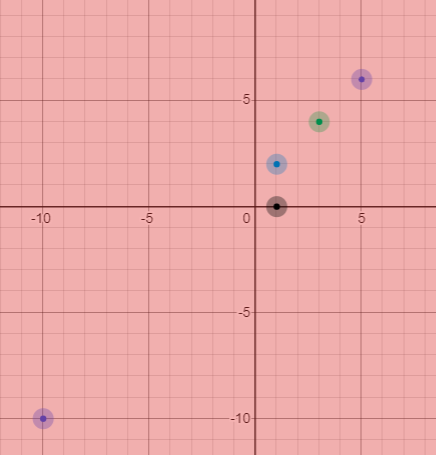# Orange Boy Can You Solve It Out? Ep.24

It's going to be the 70th birthday of China! Orange Boy is looking forward to it and to celebrate this, he became Red Boy! So he proposes the following problem for you:
On Oct.1st. There is a military parade. The Beijing city can been seen as a 2D plane and each person in the parade can be seen as a single point. There are N people in the parade and the i-th of them is located at (x_i,y_i) initally.
On each second, each person will move down for 1 unit. That's to say, if a person initally at (x,y) will be at (x,y-t) after t seconds.
Our beloved Chairman Xi is located at (0,0) with an inital sight angle of 0 degree. If he has a sight angle of D degree, he will be able to see every person in the parade in the area which can be sweeped from the positive x axis by rotating D degree. He can see through people, means his sight will not be blocked by anyone.
For example, if Xi's sight angle is 45 deg, he will see the area(including the border line) below:Now there are Q operations which you need to proceed them in a row.

1. "hmcis x" if the time is at the x-th second, how many people can Xi see? The x is not necessarily increasing.

2. "cmpov x" change the sight angle to x degree.

Proceed the queries.

# Example

people = {{1,2},{3,4},{5,6},{1,0},{-10,-10}}
query={
hmcis 0,
cmpov 45,
hmcis 2,
cmpov 360,
hmcis 0
}
Output:
3
5
Explain:
At first, Xi's sight angle is 0, so he cannot see anyone.
Then after he changes his sight angle to 45.
Then at 2nd second, the plot looks like follow:Then he changes his sight angle to 360.
Then he can see:# Constriants

Subtask 2: for all x in "cmpov x", x is a multiple of 45.
Subtask 4: for all x in "cmpov x", x is a multiple of 30.
Subtask 5: for all x in "hmcis x", x=0
Subtask 6: for all x in "hmcis x", x is increasing
Subtask 7: for all x in "cmpov x", x is a multiple of 90.
Subtask 9: 1\leq q,n\leq 10^5,1\leq |X_i|,|Y_i|\leq 10^9,X_i\in Z，Y_i\in Z. For any x in te queries, 0<=x<=1e9, x is an integer.

# Can you solve it?

THE END